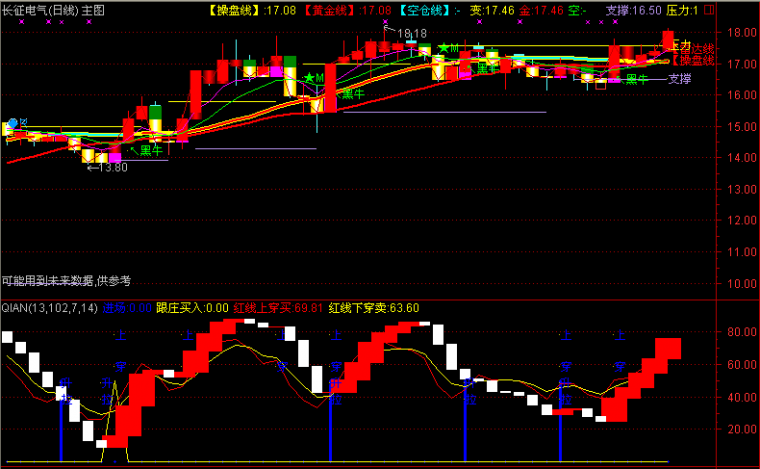﻿ 飞鱼出水-其他软件公式 －程序化交易（CXH99.COM）

# 飞鱼出水[其他软件公式]

来源:程序化99（ WWW.CXH99.COM ）

VAR1:=REF(LOW,1);
VAR2:=SMA(ABS(LOW-VAR1),3,1)/SMA(MAX(LOW-VAR1,0),3,1)*100;
VAR3:=EMA(IF(CLOSE*1.2,VAR2*10,VAR2/10),3);
VAR4:=LLV(LOW,38);
VAR5:=HHV(VAR3,38);
VAR6:=IF(LLV(LOW,90),1,0);
VAR7:=EMA(IF(LOW<=VAR4,(VAR3+VAR5*2)/2,0),3)/618*VAR6;

V1:=LLV(H,240);

V2:=HHV(H,240);

V3:=SUM(VOL,5)/CAPITAL;
CYF5:=100-(100/(1+V3));(注：由于人数限制，QQ或微信请选择方便的一个联系我们就行，加好友时请简单备注下您的需求，否则无法通过。谢谢您！)

【字体： 】【打印文章】【查看评论

没有相关内容